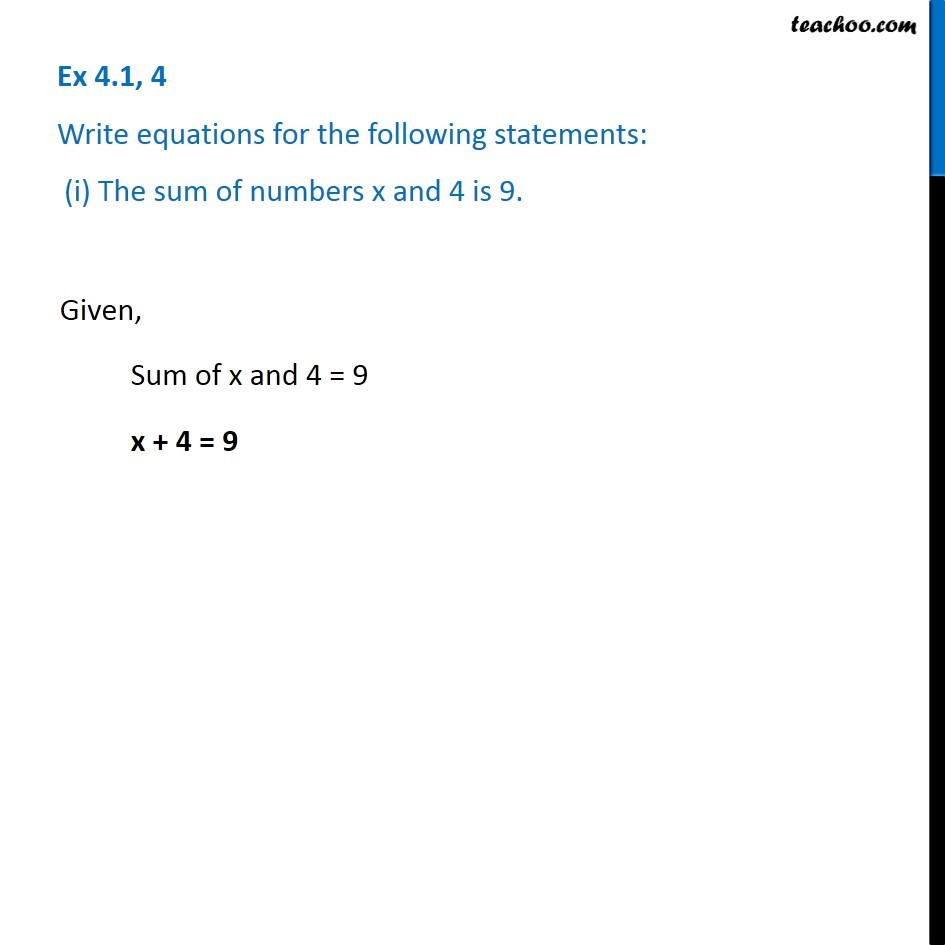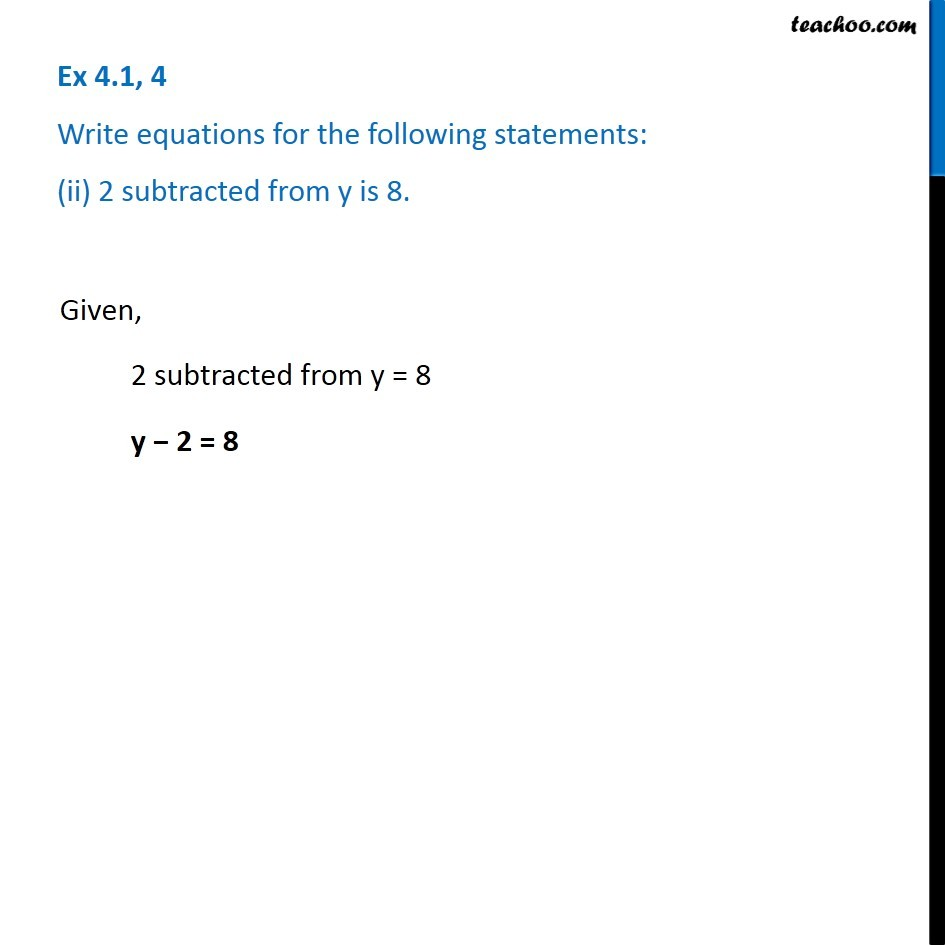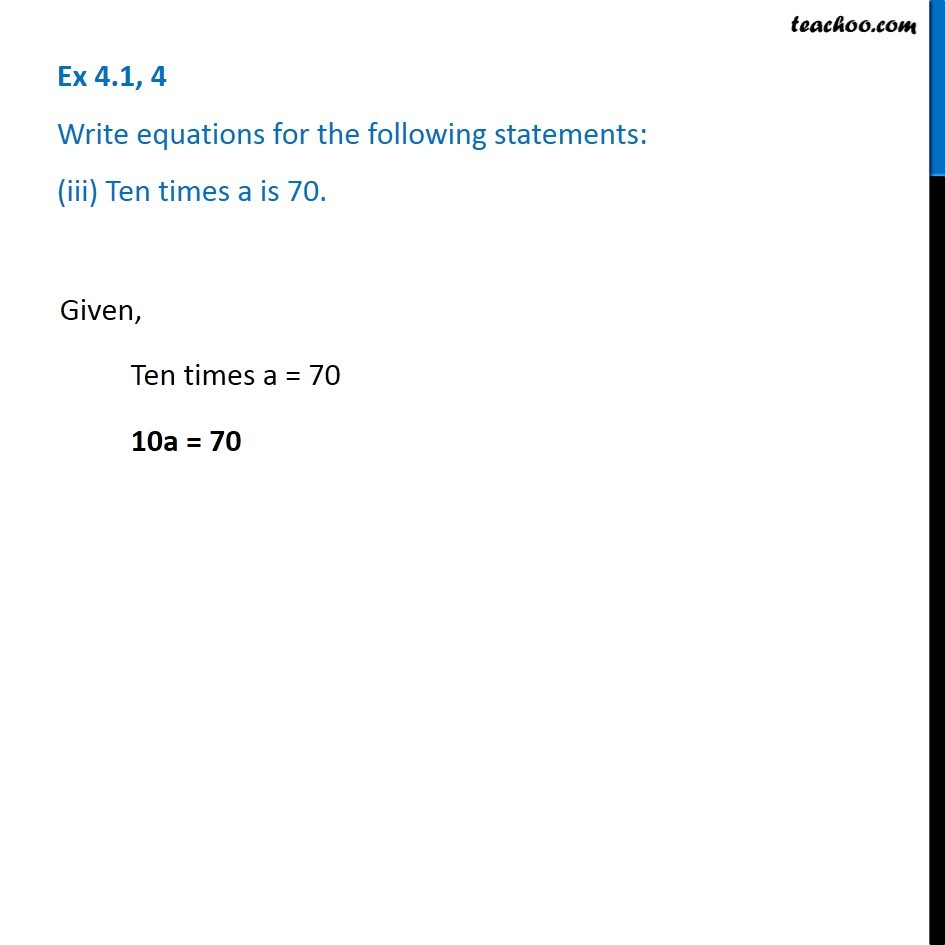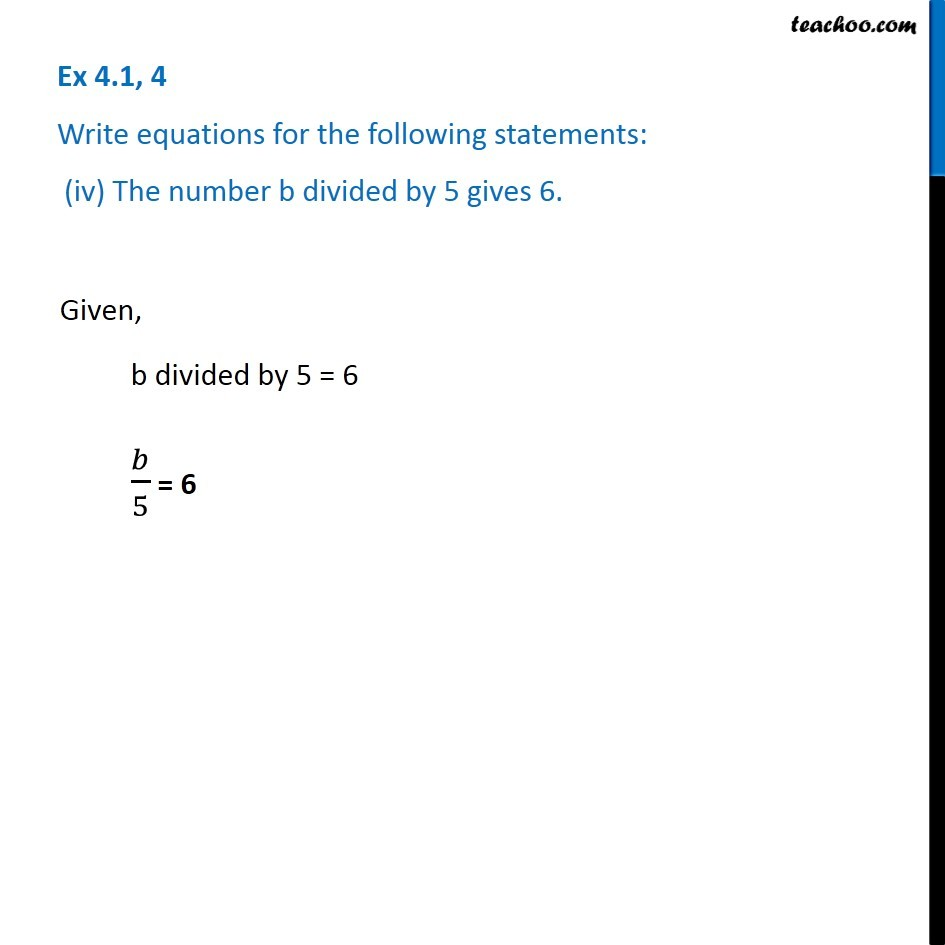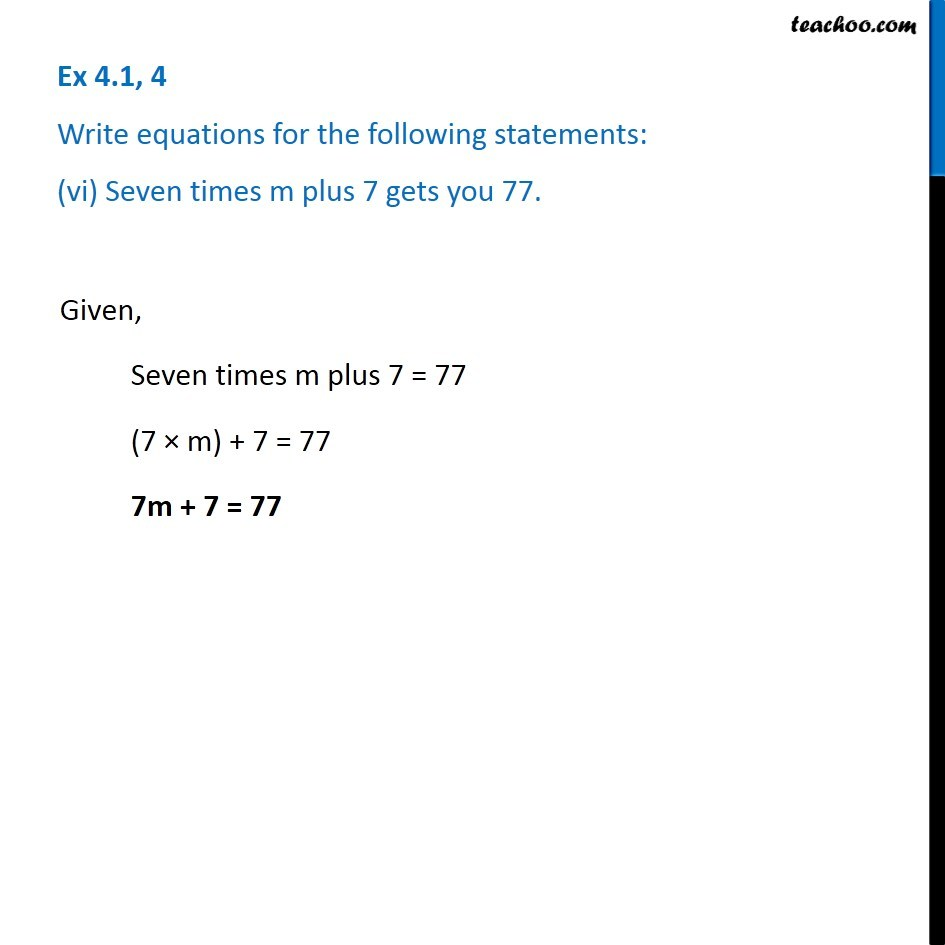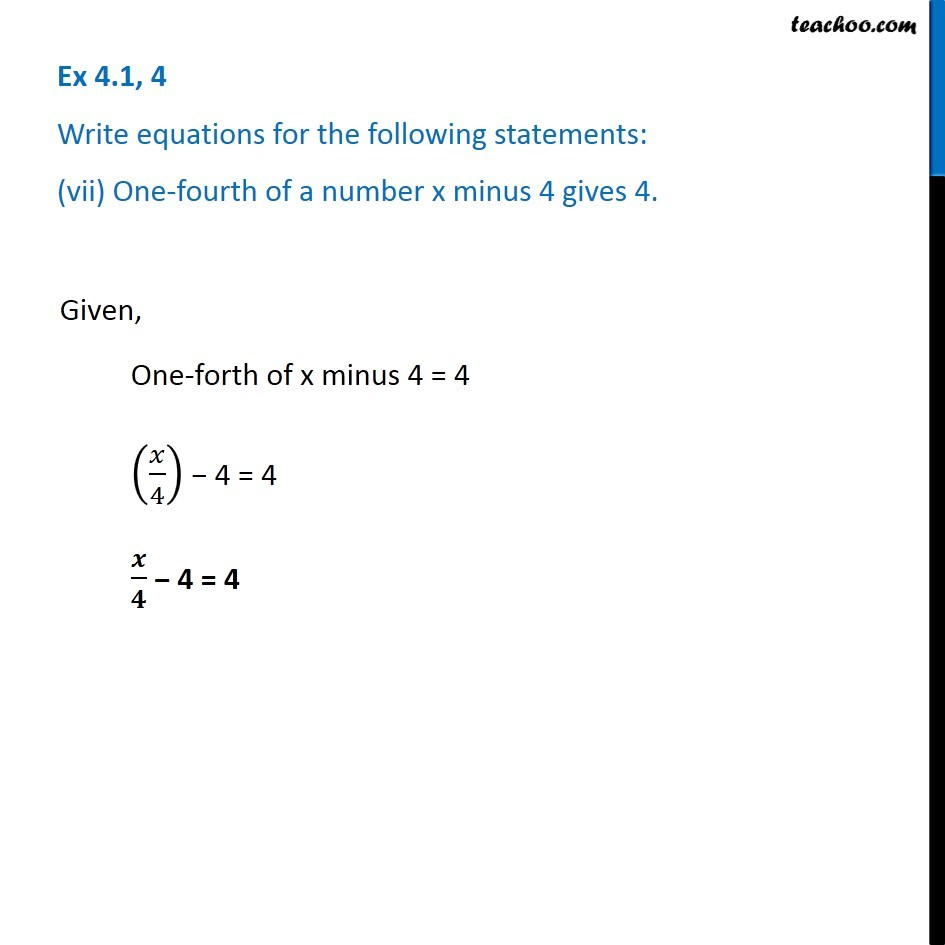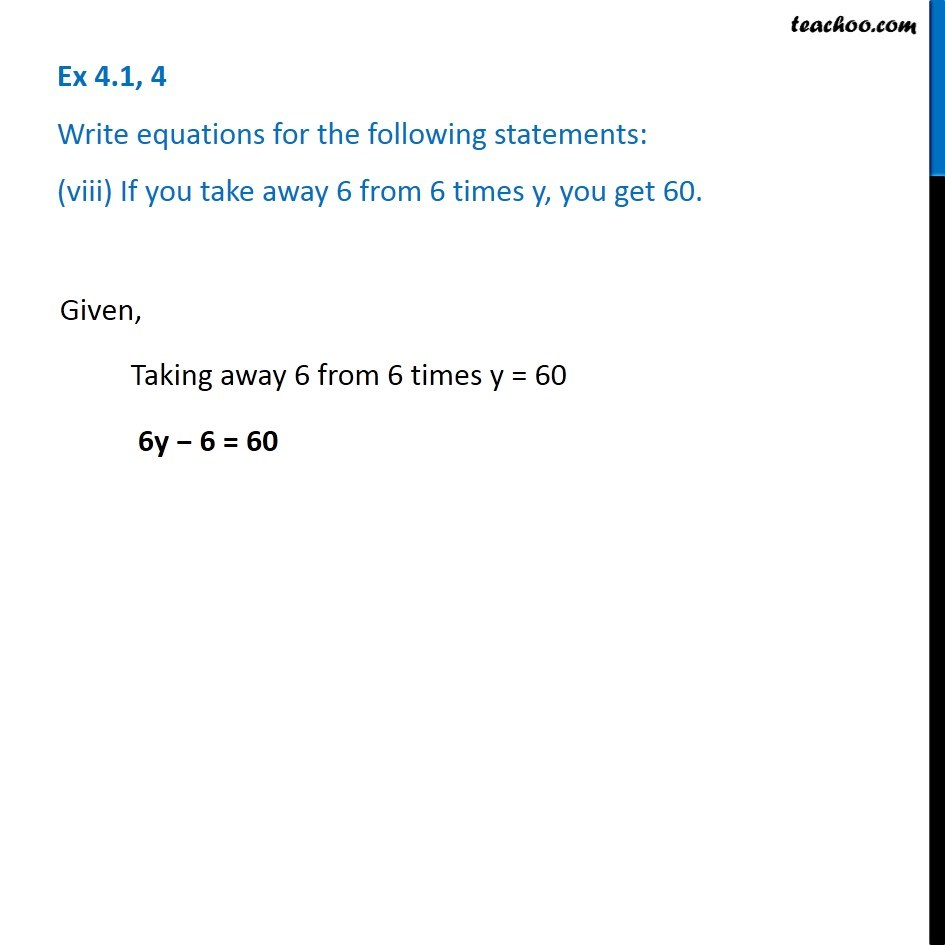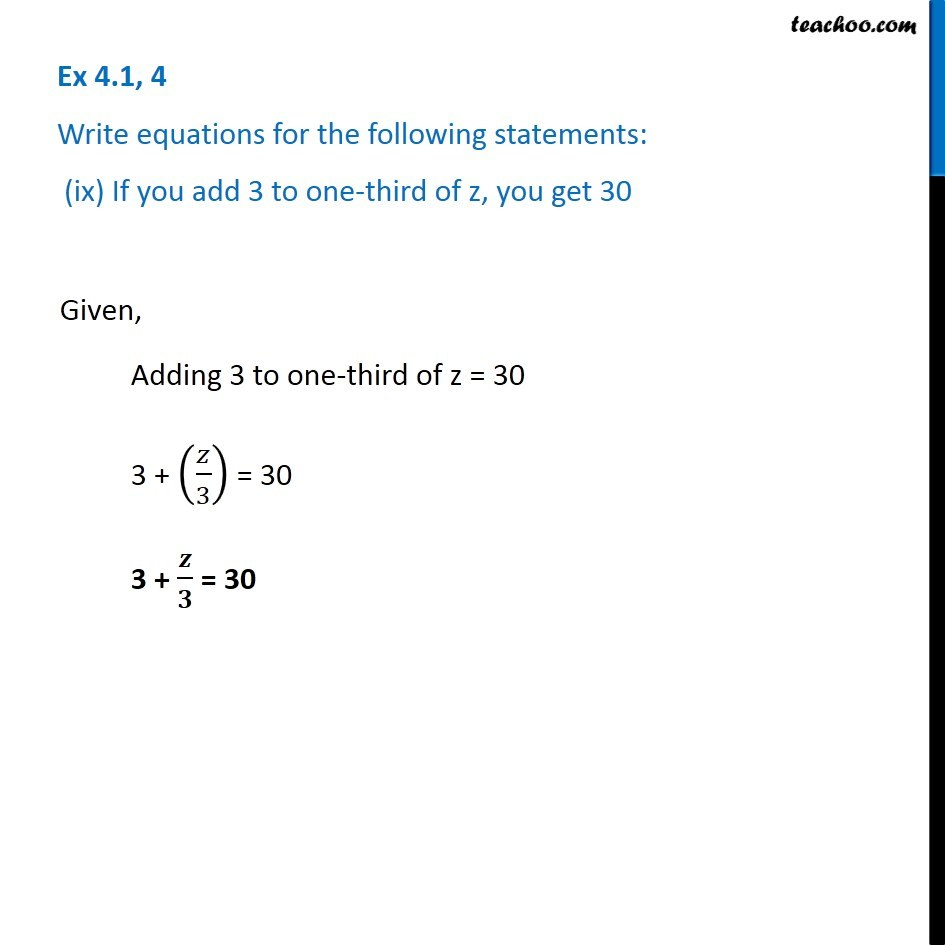1. Chapter 4 Class 7 Simple Equations
2. Serial order wise
3. Ex 4.1

Transcript

Ex 4.1, 4 Write equations for the following statements: (i) The sum of numbers x and 4 is 9.Given, Sum of x and 4 = 9 x + 4 = 9 Ex 4.1, 4 Write equations for the following statements: (ii) 2 subtracted from y is 8. Given, 2 subtracted from y = 8 y − 2 = 8 Ex 4.1, 4 Write equations for the following statements: (iii) Ten times a is 70. Given, Ten times a = 70 10a = 70 Ex 4.1, 4 Write equations for the following statements: (iv) The number b divided by 5 gives 6. Given, b divided by 5 = 6 𝑏/5 = 6 Ex 4.1, 4 Write equations for the following statements: (v) Three-fourth of t is 15. Given, forth of t = 15 3𝑡/4 = 15 Ex 4.1, 4 Write equations for the following statements: (vi) Seven times m plus 7 gets you 77. Given, Seven times m plus 7 = 77 (7 × m) + 7 = 77 7m + 7 = 77 Ex 4.1, 4 Write equations for the following statements: (vii) One-fourth of a number x minus 4 gives 4. Given, One-forth of x minus 4 = 4 (𝑥/4) − 4 = 4 𝒙/𝟒 − 4 = 4 Ex 4.1, 4 Write equations for the following statements: (viii) If you take away 6 from 6 times y, you get 60.Given, Taking away 6 from 6 times y = 60 6y − 6 = 60 Ex 4.1, 4 Write equations for the following statements: (ix) If you add 3 to one-third of z, you get 30 Given, Adding 3 to one-third of z = 30 3 + (𝑧/3) = 30 3 + 𝒛/𝟑 = 30

Ex 4.1